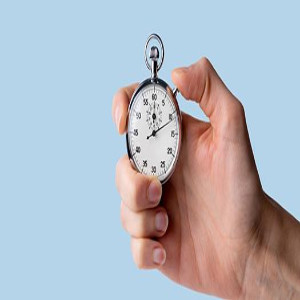Approved & Edited by ProProfs Editorial Team
At ProProfs Quizzes, our dedicated in-house team of experts takes pride in their work. With a sharp eye for detail, they meticulously review each quiz. This ensures that every quiz, taken by over 100 million users, meets our standards of accuracy, clarity, and engagement.
| Written by Logaprabha.v
L
Logaprabha.v
Community Contributor
Quizzes Created: 1 | Total Attempts: 713
Questions: 10 | Attempts: 735Settings• 1.

### What value is 8 in the number 380?

• A.

Thousands

• B.

Hundreds

• C.

Tens

• D.

Ones

C. Tens
Explanation
The value 8 in the number 380 represents the tens place. In the number 380, the digit 8 is in the second position from the right, which is the tens place. The tens place represents the quantity of tens in a number. In this case, there are 8 tens in the number 380.

Rate this question:

• 2.

### Which is a way to show 451?

• A.

40 + 50 + 1

• B.

4 hundreds + 11 tens + 50 ones

• C.

4 + 5 + 1

• D.

400 + 50 + 1

D. 400 + 50 + 1
Explanation
The correct answer is 400 + 50 + 1. This answer represents the number 451 by breaking it down into its place values. The first digit, 4, represents 400 (4 hundreds), the second digit, 5, represents 50 (5 tens), and the third digit, 1, represents 1 (1 one). By adding these place values together, we get the number 451.

Rate this question:

• 3.

### Which digit is in the hundreds place in the number 9,082?

• A.

9

• B.

0

• C.

8

• D.

2

B. 0
Explanation
The digit in the hundreds place in the number 9,082 is 0.

Rate this question:

• 4.

### Jose was counting by 5's. He stopped at 85. What number would come next?

• A.

80

• B.

86

• C.

90

• D.

95

C. 90
Explanation
The question states that Jose was counting by 5's and stopped at 85. To find the next number, we need to continue counting by 5's. Starting from 85, the next number would be 90, as we are adding 5 to the previous number each time. Therefore, the correct answer is 90.

Rate this question:

• 5.

### An office building is 3 stories tall. There are 20 offices on the first floor. There are 6 more offices on the second floor than on the first floor, and there are 2 more offices on the third floor than on the second floor. How many offices are there in all?

• A.

28

• B.

32

• C.

74

• D.

84

C. 74
Explanation
The number of offices on the first floor is 20. The number of offices on the second floor is 20 + 6 = 26. The number of offices on the third floor is 26 + 2 = 28. Therefore, the total number of offices in the building is 20 + 26 + 28 = 74.

Rate this question:

• 6.

### What ten should you round 743 to?

• A.

740

• B.

800

• C.

700

• D.

850

A. 740
Explanation
To round 743 to the nearest ten, we look at the digit in the ones place, which is 3. Since 3 is less than 5, we round down. Therefore, 743 rounded to the nearest ten is 740.

Rate this question:

• 7.

### 12+7_____15+3

• A.

<

• B.

>

• C.

=

• D.

Option 4

B. >
Explanation
The given answer ">" is correct because 12+7 is greater than 15+3. The sum of 12+7 is 19, while the sum of 15+3 is 18. Therefore, 19 is greater than 18, resulting in the ">" symbol being the correct choice.

Rate this question:

• 8.

• A.

26

• B.

38

• C.

36

• D.

40

C. 36
Explanation

Rate this question:

• 9.

### I am the biggest 3 digit number. If you add 1 to me, what do i become?

• A.

900

• B.

999

• C.

1000

• D.

1001

C. 1000
Explanation
If you add 1 to the biggest 3-digit number (999), it becomes 1000. This is because 999 is the largest number that can be formed using 3 digits (9, 9, and 9). Adding 1 to it increases the hundreds place to 1, making it 1000.

Rate this question:

• 10.

### Double 26

• A.

50

• B.

52

• C.

42

• D.

100Back to top